# Combinational Logic Circuits

Tom Kelliher, CS26

Oct. 8, 1996

# Minimization

Why bother? Speed, power, real estate.

Minimization Techniques:

1. Quine-McCluskey.

2. Karnaugh Maps.

3. Espresso.

## K Maps

1. Minimal cover.

2. The map is a sphere.

3. Don't cares in real circuits.

4. Gray code row, column numbering.

5. Converting the covers to equations.

6. Axis labels.

### K-Map Templates

Two variable:Example:Three variable: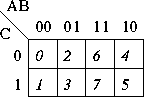Example:Four variable: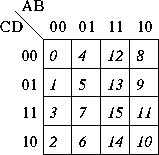Example: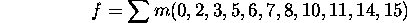Try the following:

1. The sum output of a full binary adder.

2. The carry out output of a full binary adder.

3. A circuit which compares two-bit unsigned numbers. There are three outputs: inputs equal, first greater, second greater.

# Synthesis with NAND and NOR Gates

Why do TTL and CMOS designers use these gates?

Why are ECL designers so lucky?

# Exercises

1. Design and implement a 2-1 multiplexer, using a K-map for minimization of the output equation. Here's the truth table: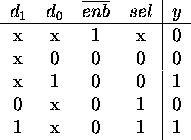A multiplexer works like a switch. One way of drawing them is: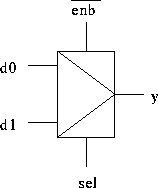2. Design and implement a circuit to take a BCD-encoded digit and drive a seven-segment display (used in watches and calculators). Use a K-map to minimize each of the seven output equations. Take advantage of don't cares. Here is the labeling for the display: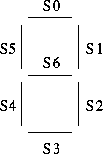3. Design and implement a circuit to take a BCD-encoded digit and increment it by one (nine should be ``incremented'' to zero). Use a K-map to minimize each of the four output equations. Take advantage of don't cares.

Thomas P. Kelliher
Mon Oct 7 09:49:09 EDT 1996
Tom Kelliher